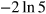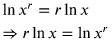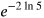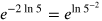1
55
views
38a
Problem

Problem 38a

Chapter 1: Functions and ModelsTextbook ExpertVerified Tutor
13 Oct 2021

Given information

Given an exponential function with exponent :Step-by-step explanation

Step 1.

First, will try to simplify the index:Recall that:Thus we can writeas shown below: